# Change Nickname

Current Nickname:
Search
v2.x
v4.0

# Data Stream | Clause

## Overview

### Clause

Besides the chain statements such as CRUD and query, the rest of a UQL statement are clauses begin with keywords. Clauses can compute and process the results of the preceding statements, and pass the new results to subsequent statements or return the results. This chapter will introduce the following clause keywords:

• GROUP BY
• ORDER BY
• SKIP
• LIMIT
• WHERE
• RETURN
• WITH
• UNCOLLECT

The query results of UQL are passed between different chain statements and clauses in the form of data stream, and eventually returned to user.

### Data Stream

Each query, insertion, update chain statement and clause in UQL produces data stream, and its length (the number of rows of the data stream) is the number of the queried or processed results; multiple data columns derived from one data stream which is produced by the same statement are called homologous columns. In the vast majority cases, the lengths of homologous columns are the same, but there are rare instances (aggregation, deduplication, UNCOLLECT, etc.) where the lengths of homologous columns are different.

Example: The template query statement in the image below found 5 paths; the columns of the end nodes of the paths, the column of the length (number of edges) of the paths, as well as the column of paths are homologous columns, and each contains 5 pieces of data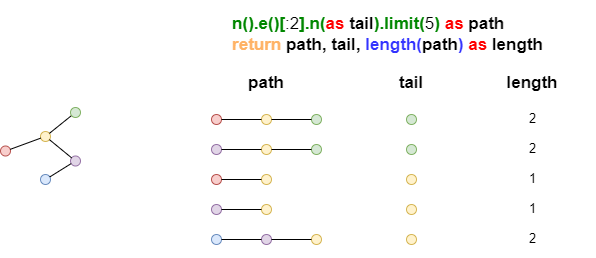If one of the homologous columns is aggregated, deduplicated (column length becomes smaller) or processed with UNCOLLECT operation (column length becomes larger), this may affect the length of other homologous columns, details to follow in the latter sections of WITH and RETURN.

When data columns come from data streams that are produced by different statements, they are non-homologous columns, even under the circumstances when they are of the equal length.

Example: The two K-Hop query statements in the image below produce two data streams of lengths 3 and 5, these two columns of data are non-homologous columns.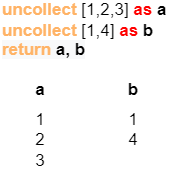#### Data Stream and Chain Statement

During the execution of UQL, when the data stream produced earlier is used as the input to the subsequent chain statement, it can be comprehended as this, the times the chain statement are executed equals to the length of that data stream, and each execution uses one row of data in the data stream (system will apply some optimizations based on the actual situation).

Example: The template query statement in the image below found multiple paths, with 4 end nodes after dedepulication, it can be viewed that the deletion statement afterwards is executed 4 times in total, and deletes 1 node each time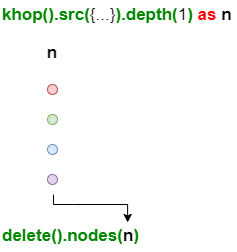A query statement, which is executed multiple times according to the length of the data stream, would produce a new data stream and its length equals to the sum of the number of results of each query.

Example: The template query statement in the image below found 2 nodes - blue and red, the first execution of the subsequent node query statement found 2 blue nodes, the second execution found 3 red nodes, that's 5 pieces of data in total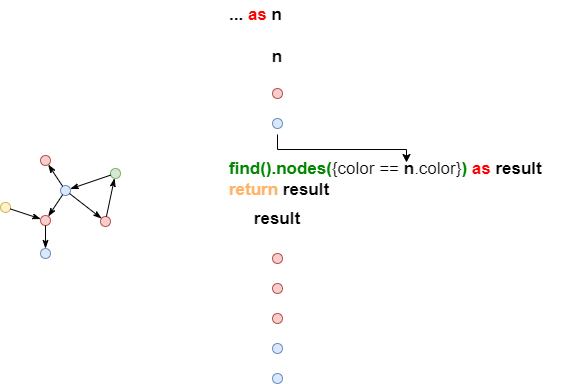When multiple data streams are simultaneously used as the input to a chain statement, it can be understood that the number of times the chain statement executed equals to the length of the shortest stream.

Example: The template query statement in the image below produced two data streams of length 3 and 2, the longer data stream is trimmed to the shortest length of 2, eventually 2 rows of data are passed into the third query statement and 3 query results are obtained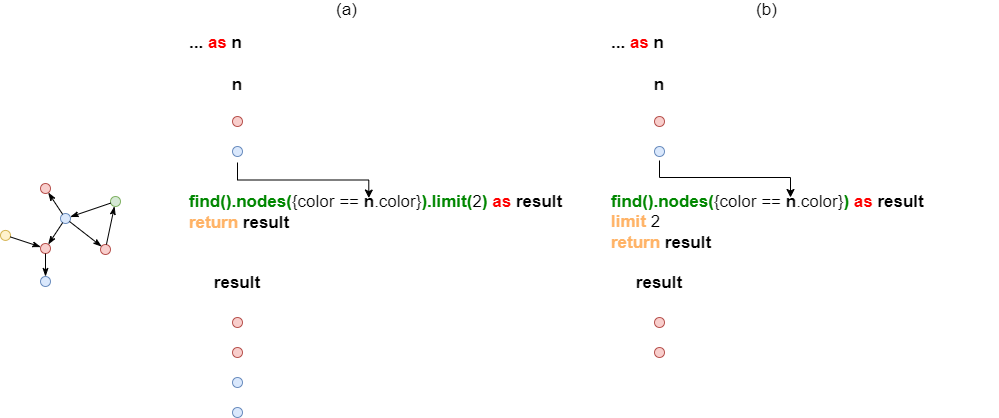This kind of operation of trimming multiple data streams to the shortest length is not very useful in practical applications; more often, multiple data streams are to be processed into one data stream, in order to be used in the subsequent chain statement, details to follow in the latter section of WITH.

#### Data Stream and Clause

Different clauses work differently when processing data streams. Some clauses are only able to process one stream, such as GROUP BY, ORDER BY, LIMIT and SKIP; while other clauses can process multiple streams, such as WITH, RETURN and WHERE. Whether it's one or multiple streams are processed, all clauses produce one new stream, and the various columns of data in this stream are homologous columns. See the following detailed introduction to each clause.

## GROUP BY | Grouping

GROUP BY clause can divide the rows of data in a data stream into multiple groups; in each group, keep the first row of data and discard the rest rows; when being used with aggregation operation functions, place the aggregated value produced by each group in the first row and discard the other rows.

Syntax: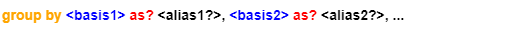where

• `<stream>` is the query statement or clause that outputs a data stream, the data stream is the grouping object of GROUP BY;
• Grouping basis `<basis>` can be the reference of alias (and its mapping class functional operations, numerical operations) in `<stream>`, custom alias is supported;
• Multiple `<basis>` will be referred from left to right to achieve multi-level grouping.

Example: The two query statements in the image below produced two data streams, both of length 7; followed by GROUP BY clause that divides the second data stream into 4 groups based on the shape of start nodes and the color of end nodes in the paths; the final outputs are two data streams, the length of the first is 7 and the second is 4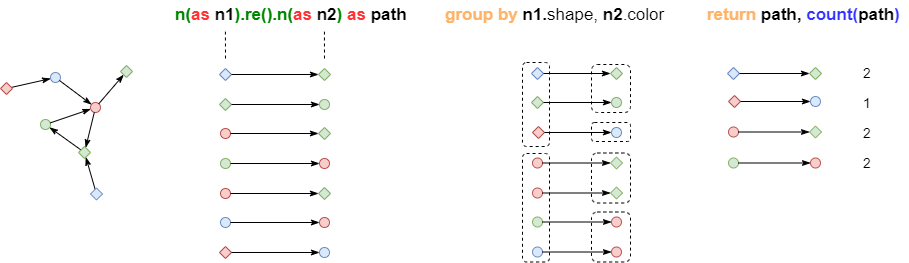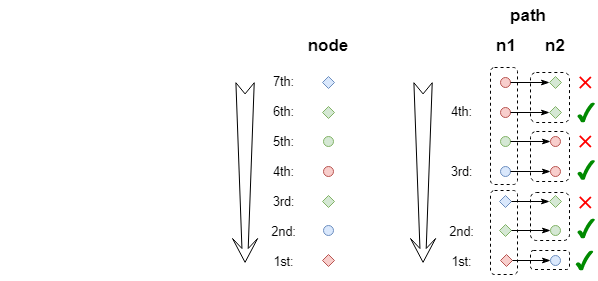Example: Group cards by card level, return the total number of cards at each level

``````find().nodes({@card})
group by nodes.level as level
return level, count(nodes)
``````

Example: Find cards held by accounts U001, U002, U003, and return the array of cards held by each account

``````n({_id in ["U001","U002","U003"]} as accounts)
.re({@own}).n({@card} as cards)
group by accounts as holder
return holder, collect(cards._id)
``````

Example: Count each level of cards held by each level of accounts, return account level, card level and the count of cards

``````n({@account} as accounts)
.re({@own}).n({@card} as cards)
group by accounts.level as a, cards.level as c
return a, c, count(cards)
``````

## ORDER BY | Sorting

ORDER BY can adjust the order of rows in a data stream.

Syntax: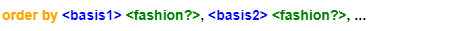where

• `<stream>` is the query statement or clause that outputs a data stream, the data stream is the sorting object of ORDER BY;
• Sorting basis `<basis>` can be the reference of alias (and its mapping class functional operations, numerical operations) in `<stream>`, only numeric value, string and date/time are supported;
• Multiple `<basis>` will be referred from left to right to achieve multi-level sorting;
• Sorting method `<fashion>` is `asc` (default) or `desc`, case insensitive.

Example: The two query statements in the image below produced two data streams of unequal length; followed by ORDER BY clause that sorts the second data stream by ascending radius and descending UUID of the end nodes in the path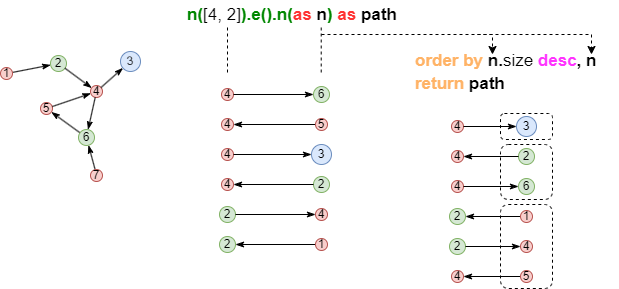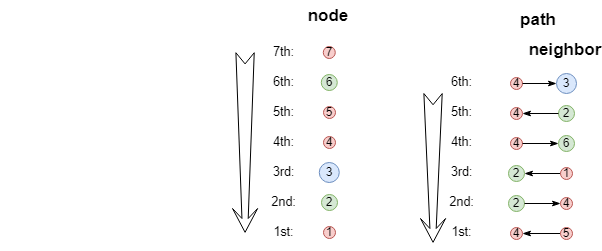Example: Find the top 10 cards with the largest balance

``````find().nodes({@card})
return nodes limit 10
order by nodes.balance desc
``````

Example: Find cards that received direct transactions from C001, deduplicate and return these cards with card level in the ascending order

``````n({_id == "C001"}).re({@transaction}).n({@card} as cards)
return distinct(cards)
order by cards.level asc
``````

Example: Count cards in each card level and return the top 3 levels with the largest number of cards

``````find().nodes({@card})
group by nodes.level as level
return level, count(nodes) as total limit 3
order by total desc
``````

Example: Find acount U001's 1-step outbound transactions, return these transaction paths sorted by descending transaction amount, and recipient cards sorted by ascending card level

``````n({_id == "U001"}).e({@own}).n({@card})
.re({@transaction} as trans).n({@card} as cards)
return trans, cards
order by trans.amount desc, card.level
``````

## SKIP | Skip

SKIP can discard the first N rows of data in a data stream.

Syntax: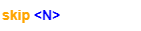where:

• `<stream>` is the query statement or clause that outputs a data stream, the data stream is the operation object of SKIP;
• `<N>` is the number of rows to discard, it's a non-negative integer.

Example: The two query statements in the image below produced two data streams, both of length 5; followed by SKIP clause that discards the first 3 rows of the second data stream; the final outputs are two data streams, the length of the first is 5 and the second is 2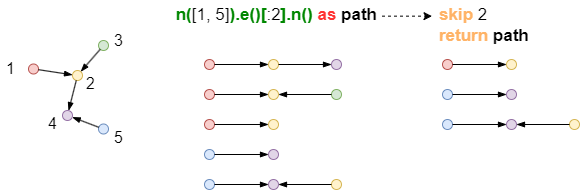Example: Find cards that received transactions from C001, return cards from the 4th row of data sorted by the descending balance; that is, don't return the 3 cards with the largest balance

``````n({_id == "C001"}).e({@transaction}).n({@card} as cards)
return cards skip 3
order by cards.balance DESC
``````

## LIMIT | Limit Length

LIMIT can keep the first N rows of data in a data stream, and discard the rest rows.

Syntax: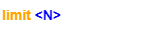where

• `<stream>` is the query statement or clause that outputs a data stream, the data stream is the operation object of LIMIT;
• `<N>` is the number of rows to keep if it's a non-negative integer, it keeps all rows of data when it's -1.

Example: The two query statements in the image below produced two data streams, both of length 5; followed by LIMIT clause that keeps the first 3 rows of the second data stream; the final outputs are two data streams, the length of the first is 5 and the second is 3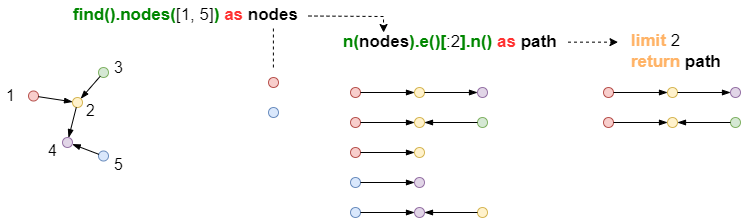Except for the `find()` command, other query commands support the use of parameter `limit(<N>)` to limit the number of the output results of a single execution; while LIMIT clause is to limit the length of the output results produced by the preceding statement after multiple executions. Please note the difference between these two.

Example: Find cards that received transactions from C001, return 10 cards sorted by descending balance; that is, to return the 10 cards with the largest balance

``````n({_id == "C001"}).e({@transaction}).n({@card} as cards)
with distinct (cards)
order by cards.balance DESC
return cards limit 10
``````

Analysis: When both `limit` and `order by` appear after `return`, `order by` is always executed pripor to `limit`

Example: Randomly find 10 cards that received transactions from C001, return cards sorted by the descending balance

``````n({_id == "C001"}).e({@transaction}).n({@card} as cards)
with distinct (cards)
limit 10 return cards
order by cards.balance DESC
``````

## WHERE | Check Row by Row

WHERE can discard rows of data in one or multiple data streams that don't meet the conditions, and combine the rows of data that meet the conditions into a data stream.

Syntax: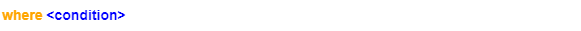where

• `<stream>` is the query statement or clause that outputs different data streams, these data streams are the operation objects of WHERE;
• Judgement condition `<condition>` is made up of the reference of alias (and its mapping class functional operations, numerical operations) in `<stream>`; when `<condition>` is a conditional expression, rows that are "false" will be discarded; when `<condition>` is a query statement, rows with no query result will be discarded.

WHERE can disassemble some filtering conditions from Ultipa filter to improve the readability of the statement; it can also implement complex subgraph query equivalent to the `graph()` command.

Example: The two query statements in the image below produced two data streams of unequal length; followed by WHERE clause that keeps the 3rd row according to the judgement condition, the length of the final combined data stream is 1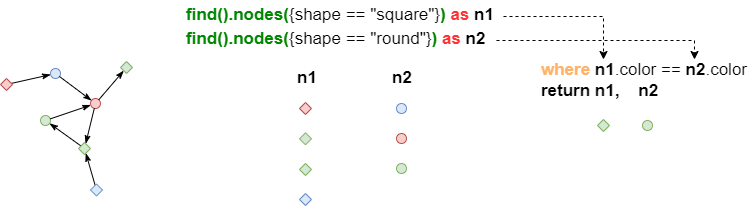### Insert Conditional Expression

Example: Use WHERE clause to compare the balance between C001 and its neighbor cards, upgrade those neighbor cards that have higher balance than C001 to the next upper level

``````khop().src({_id == "C001"} as C001).depth(1).node_filter({@card}) as neighbors
where neighbors.balance > C001.balance
update().nodes({_id == neighbors._id}).set({level: this.level + 1})
``````

Note: Template query statement can also be used in this example to define neighbors, in that case the comparison of balance can be put in the filter of node template.

Example: Find 3-step transaction paths from C001 to C002, it's required that at least one of the the intermediate cards A and B to have a level of 5

``````n({_id == "C001"}).e().n({@card} as cardA)
.e().n({@card} as cardB)
.e().n({_id == "C002"}) as paths
where cardA.level == 5 || cardB.level == 5
return paths
``````

### Insert Query Statement (Under Development)

Example: C001 transfered to C002 through agent cards, find those agent cards that are also 2-hop neighbors of C003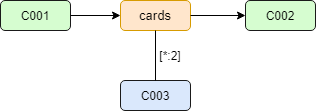``````n({_id == "C001"}).re().n({@card} as agent).re().n({_id == "C002"})
where n({_id == agent._id}).e()[*:2].n({_id == "C003"})
return agent
``````

Note: WHERE clause further filters the "agent" in the data stream to find out whether the 2-step shortest path to C003 exists for each "agent"; if so, passes the "agent" to the RETURN clause that follows.

Calling alias in WHERE clause and doing search is similar to `graph()` command which finds the intersection of common alias in multiple template query results. For instance, the above example can be rewritten with a subgraph template as follows:

``````graph([
n({_id == "C001"}).re().n({@card} as agent).re().n({_id == "C002"}),
n({_id == agent._id}).e()[*:2].n({_id == "C003"})
])
return agent
``````

## RETURN | Return

RETURN can perform functional operations on a data stream, or combine multiple data streams into a single data stream; when combining, it first trims every data stream to the length of its own aggregated column (if has), then trims all streams to the length of the shortest data stream.

Syntax: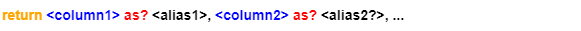where

• `<stream>` is the query statement or clause that outputs different data streams, these data streams are the operation objects of RETURN;
• The returned value `<column>` is the reference of alias (and its mapping class functional operations, numerical operations) in `<stream>`, custom alias is supported.

Example: The two query statements in the image below produced two data streams of unequal length; followed by RETURN clause that combines the two data streams, the length of the final combined data stream is 3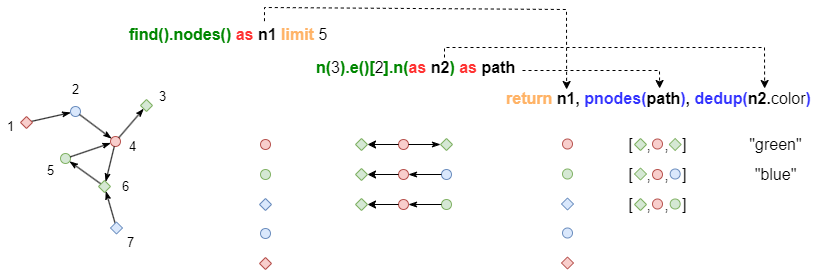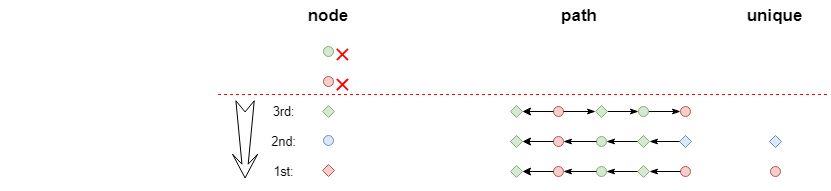In RETURN clause, deduplication operation of a certain column doesn't affect the length of the data stream it's in.

Read chapter Query for the meaning of alias calls in different formats in RETURN clause.

Example: Find 10 @card or @account nodes, return nodes with card balance

``````find().nodes({@card || @account})
return nodes{balance} limit 10
``````

Note: The query above returns two kinds of node @card and @account, the returned accounts have empty value since balance is not a property of @account.

Example: Find 10 @card or @account nodes, return nodes with time

``````find().nodes({@card || @account})
return nodes{time} limit 10
``````

Note: This query also returns two kinds of node @card and @account, the returned cards and accounts both have time values since time is a property of both scheams.

Example: Find 10 edges, return edges with the names of their schemas

``````find().edges()
return edges.@ limit 10
``````

Example: Find all property values of 10 nodes

``````find().nodes()
return nodes{*} limit 10
``````

Example: Find the recpient cards of C001's 2-step outbound transaction by K-Hop query, only return the card numbers

``````khop()
.src({_id == "C001"}).depth(2)
.node_filter({@card})
.edge_filter({@transaction})
.direction(right) as nodes
return nodes._id
``````

Example: Return 10 paths from C001 to C002 with 3 steps, return UUID of nodes and edges

``````ab()
.src({_id == "C001"}).dest({_id == "C002"}).depth(3)
.direction(right) as paths
return paths limit 10
``````

Example: Return transition paths from C001 to C002 with 3 steps, with card number and transation amount

``````ab()
.src({_id == "C001"}).dest({_id == "C002"}).depth(3)
.node_filter({@card})
.edge_filter({@transaction})
.direction(right) as paths
return paths{_id}{amount}
``````

Example: Return 10 paths from C001 to C002 with 3~5 steps, with all node properties and edge's time property (if has)

``````ab()
.src({_id == "C001"}).dest({_id == "C002"}).depth(3:5) as paths
return paths{*}{time} limit 10
``````

Example: Return 10 paths from C001 to C002 with 3~5 steps, with all node properties and edge properties

``````ab()
.src({_id == "C001"}).dest({_id == "C002"}).depth(3:5) as paths
return paths{*} limit 10
``````

Example: Count the number of paths from C001 to C002 with 3 steps

``````ab()
.src({_id == "C001"}).dest({_id == "C002"}).depth(3) as paths
return count(paths)
``````

## WITH | Pass

WITH can perform functional operations on a data stream, or combine multiple data streams into a single data stream; when combining, it first deduplicates the entire row based on its own deduplication column (if has) in every data stream, and trims every data stream to the length of its own aggregated column (if has), then combines all streams by with Cartesian product.

`Syntax` of WITH: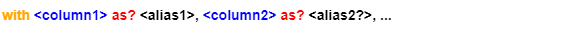where

• `<stream>` is the query statement or clause that outputs different data streams, these data streams are the operation objects of WITH;
• Passing value `<column>` is the reference of alias (and its functional operations and numerical operations) in `<stream>`, custom alias is supported.

The passing value in WITH can be used by any subsequent statement, please only use WITH clause when necessary to ensure a higher performance.

Example: The two query statements in the image below produced two data streams of unequal length; followed by WITH clause that combines the two data streams; the second data stream is first deduplicated by row and the length becomes 2, then it's combined with the first data stream by Cartesian product, the length of the final combined data stream is 3*2 = 6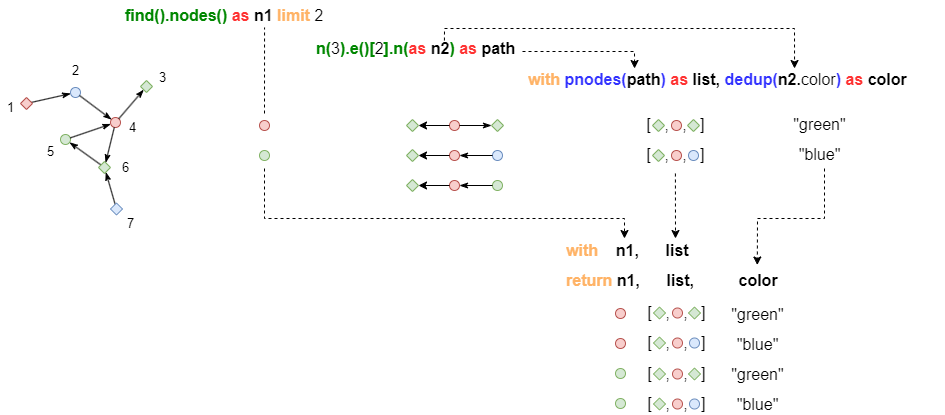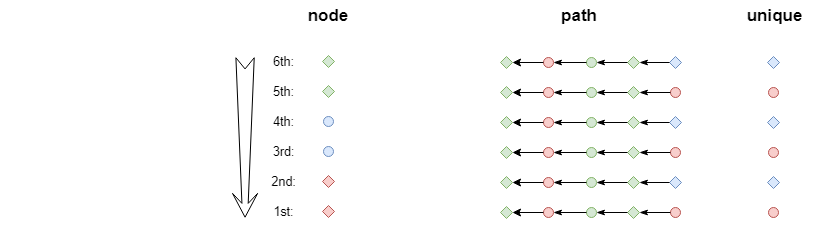In WITH clause, deduplication operation of a certain column does affect the length of the data stream it's in.

### Example: Homologous Column (Single Stream)

Example: Check all phone numbers that used in the applications within January 2021 (phone is a property of card), return the cards applied by these phone numbers

``````find().nodes({@card.time <> ["2021-01-01 00:00:00.000000","2021-02-01 00:00:00.000000"]}) as cards
with distinct(cards.phone) as phoneNO
find().nodes({@card.phone == phoneNO}) as allCards
return allcards
``````

Example: Find the highest level cards of account U001, combine the ID of these cards into an array and retrun the array

``````n({_id == "U001"}).e().n({@card} as cards)
with max(cards.level) as maxLV
n({_id == "U001"}).e().n({@card.level == maxLV} as maxLVCards)
return collect(maxLVCards._id)
``````

Example: Modify the example above, find the highest level cards of all accounts, return these accounts and array of the ID of these cards

``````n({@account} as accounts).e().n({@card} as cards)
group by accounts._id
with accounts._id as accountID, max(cards.level) as maxLV
n({_id == accountID}).e().n({@card.level == maxLV} as maxLVCards)
return accountID, collect(maxLVCards._id)
``````

Note: In this example, before the WITH clause, the data stream is grouped by accounts._id; in the WITH clause, the maximum card levels of each group are kept; the two custom aliases accountID and maxLV are used in the subsequent query statement and return clause.

Example: Find one holder of each card level, return 5 pieces of data

``````n({@account} as accounts).e().n({@card} as cards)
with accounts, distinct(cards.level) as cardLV
return accounts, cardLV limit 5
``````

Note: The two data columns accounts and cards that enter the WITH clause are homologus, cards is deduplicated by its property level and the repeated values are to be discarded, and that's when their corresponding (i.e. at same row) values in accounts are discarded too. The result is that the final returned accounts are still corresponded with card levels. If to replace WITH with RETURN in this example and write in the way as below, it's likely that there is no correspondence between the returned 5 accounts and card levels.

``````n({@account} as accounts).e().n({@card} as cards)
return accounts, distinct(cards.level)
``````

### Examples: Non-Homologous Column (Multiple Streams) - Cartesian Product Combination

Example: Find @card which property riskLevel is 3 or above, find @tag which property type is "risk", insert @has edge(s) from these cards to the "risk" tag

``````find().nodes({@card.riskLevel >= 3}) as riskCard
find().nodes({@tag.type == "risk"}) as riskTag
with riskCard, riskTag
insert().into(@has).edges({_from: riskCard._id, _to: riskTag._id})
``````

Note: In this example, assuming that the number of riskCard is N, and the number of riskTag is 1, then N*1 edges are finally inserted. If removes the WITH caluse in this example, then only 1 edge is inserted, the riskCard that exceeds the number of riskTag would be discarded.

Example: Modify the example above, give value that equals to the product of the card's riskLevel and its holder's riskCoef to the property value when inserting the @has edge(s).

``````n({@account} as owner).e({@own}).n({@card.riskLevel >= 3} as riskCard)
find().nodes({@tag.type == "risk"}) as riskTag
with owner, riskCard, riskTag
insert().into(@has).edges({_from: riskCard._id, _to: riskTag._id, value: riskCard.riskLevel * owner.riskCoef})
``````

Note: In this example, owner and riskCard are homologous, so they don't do Cartesian product; these two columns and riskTag are non-homologous, Cartesian prodouct is performed thus the number of final inserted edges is still N*1.

### Syntheses: Multi-level WITH

Example: Find cards with the highest risk level of account U001, U002 and U003, find @tag which type is "risk", insert @has edge from these cards to "risk" @tag

``````n({_id in ["U001", "U002", "U003"]} as owner).e({@own}).n({@card} as cards)
group by owner
with owner, max(cards.riskLevel) as maxRisk
n({_id == owner._id}).e({@own}).n({@card.riskLevel == maxRisk} as topRiskCards)
find().nodes({@tag.type == "risk"}) as riskTag
with topRiskCards, riskTag
insert().into(@has).edges({_from: topRiskCards._id, _to: riskTag._id})
``````

Note: In this example, the second template query command calls an alias in the first WITH clause, the number of its executions is the length of the stream outputted by the first WITH clause; `find()` command doesn't call any alias defined earlier, so it's only executed once.

Example: Modify the example above, put `find()` in front of the first WITH

``````n({_id in ["U001", "U002", "U003"]} as owner).e({@own}).n({@card} as cards)
group by owner
find().nodes({@tag.type == "risk"}) as riskTag
with owner, max(cards.riskLevel) as maxRisk
n({_id == owner._id}).e({@own}).n({@card.riskLevel == maxRisk} as topRiskCards)
with topRiskCards, riskTag
insert().into(@has).edges({_from: topRiskCards._id, _to: riskTag._id})
``````

Note: The execution result of the rewritten statement is the same with the original statement. After rewriting, the alias riskTag is not called by the WITH clause immediately after it is defined, but separated by some other WITH clauses and query commands. If there are many irrelevant clauses and commands spaced between defining the alias and referencing the alias, the reference may fail.

## UNCOLLECT | Expand

UNCOLLECT can expand all elements in arrays in a column of a data stream, the expanded elements are aligned row by row and form a new column, i.e. the length of the new column is the sum of all lengths of the original arrays. Each row of data from other homologous columns in the data stream is combined with the array elements that are released from the respective row by Cartesian product.

Syntax I: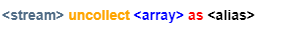where

• `<stream>` is the query statement or clause that outputs a data stream, the data stream is the operation objects of UNCOLLECT;
• `<column>` is the reference of alias (and its mapping class functional operations, numerical operations) in `<stream>`, custom alias is needed.

Syntax II:where

• `<array>` is manually defined array, custom alias is needed.

Example: The two query statements in the image below produced two data streams, of length 5 and 2; followed by UNCOLLECT clause that expands one of the array of column in the second data stream to a column of numeric values, the length of the expanded two data streams are 5 and 6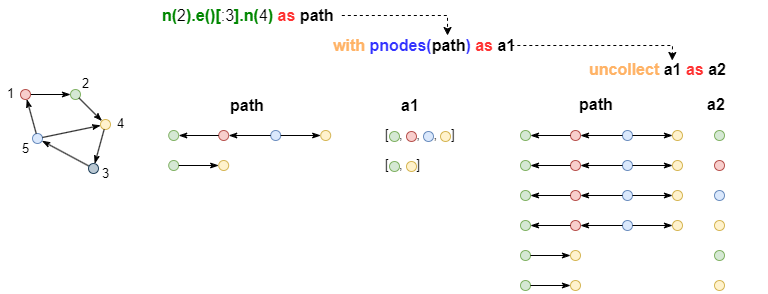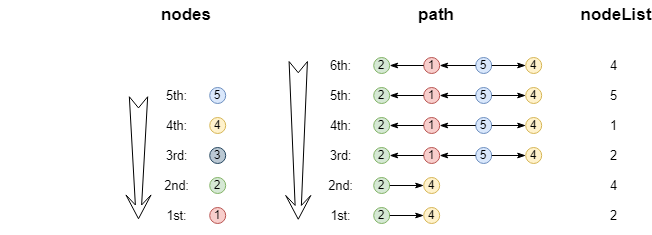Example: Find paths from node 1 to node 3 within 2 steps, deduplicate the nodes in these paths and return 10 nodes in it

``````ab().src({_uuid = 1}).dest({_uuid = 3}).depth(:2) as paths
with pnodes(paths) as nodes
uncollect nodes as nodeArray
return distinct(nodeArray) limit 10
``````

Example: Combine start node [1,2,3] and end node [4,5,6] in pairs and insert one @defult edge for each pair

``````uncollect [1,2,3] as nodes1
uncollect [4,5,6] as nodes2
with nodes1, nodes2
insert().into(@default).edges({_from_uuid: nodes1, _to_uuid: nodes2})
``````